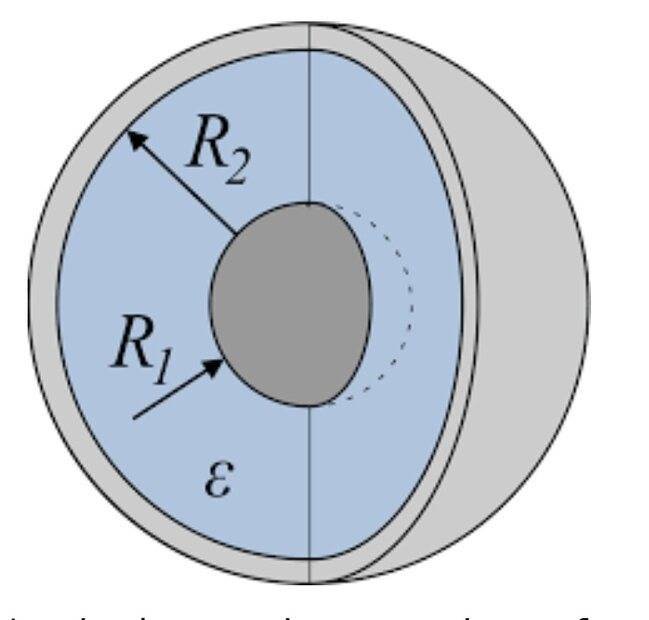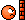# Capacitors in parallel or series

vcsharp2003
Homework Statement:
Every textbook has a very simple formula to determine equivalent capacitance when multiple capacitors are connected in parallel or series.

My question is: Does the formula apply to only parallel plate capacitors or capacitors of any shape/size?
Relevant Equations:
##\dfrac {1} {C_{eq}}= \dfrac {1} {C_1} + \dfrac {1} {C_2} + \dfrac {1} {C_3} + ...##, when capacitors are connected in series

##C_{eq}={C_1} + {C_2} + {C_3} + ...##, when capacitors are connected in parallel
To me it seems like the formula applies to capacitors of any shape or size, since textbooks never mention any limitations on capacitor type when stating these formulae.

cmb
Any.

It is the capacitances that add, not the components/devices.

If you physically construct a load of capacitor devices together you might also find there is an inter-component and/or inter-connection capacitance that is created too, and you might need to add that in as well to make sense of the result (especially RF circuits).

•DaveE, berkeman and vcsharp2003
vcsharp2003
Any.

It is the capacitances that add, not the components/devices.

If you physically construct a load of capacitor devices together you might also find there is an inter-component and/or inter-connection capacitance that is created too, and you might need to add that in as well to make sense of the result (especially RF circuits).

Can we connect a parallel plate capacitor with a spherical capacitor in series or parallel? Never seen such examples in Physics textbooks, so was just curious.

cmb
Of course.

But also (of course) you can't take one plate of a parallel plate and one sphere of a spherical capacitor and think there would be the sum of capacitances between them if held nearby each other, that would make up a different device. (I presume that might not needs saying, but just in case.)

Consider each capacitor to be a black box with two terminals. Makes no difference what is inside. But if you then open up the box then it wouldn't make the same capacitor if combined with the insides of another black box.

Some capacitors are better or worse at certain jobs, specifically they may become less capacitive and possibly even inductive as the frequency of an AC signal (or dV/dt of a transient) on them changes.

•vcsharp2003
Homework Helper
Gold Member
@cmb are you talking about how closely positioned capacitors can interact via their fringing fields? yes ok I guess this type of interaction isn't negligible in extremely high frequencies and it depends on how each capacitor is internally designed.

cmb
I'm saying that physically 'real' capacitors will have inductance and a self resonance. There is no such thing as a 'perfect' pure capacitor, they have to have conductors to route the charge in and out, which adds inductance. Even a vacuum capacitor. Some capacitors, such as electrolytics, have significant inductance at very low frequency as well as big cyclic losses (low Q/high loss coefficient/high ESR) and are completely unsuitable for high frequency work, being used instead to stabilise DC voltages.

There is far far more to a capacitor than just its capacitance.

I mean, another effect, for example, is its power rating, or VAr (reactive power handling). If you push a capacitor beyond its VAr rating it may become substantially NOT a capacitor. So also depends on how it is used.

There are also voltage limits for use, not just arcing across the terminals and across the charged plates, but the dielectric in the capacitor itself will be non-linear to higher or lower electric fields (excludes vacuum capacitors, hence their use for high power RF).

vcsharp2003
But also (of course) you can't take one plate of a parallel plate and one sphere of a spherical capacitor and think there would be the sum of capacitances between them if held nearby each other, that would make up a different device. (I presume that might not needs saying, but just in case.)

So one could say that a spherical capacitor and a parallel plate capacitor cannot be connected in series or parallel so that the formula of equivalent capacitance applies. Is that correct?

Last edited:
Homework Helper
Gold Member
So one could say that a spherical capacitor and a parallel plate capacitor cannot be connected in series or parallel so that the formula of equivalent capacitance applies. Is that correct?
Back to why not? Each of the capacitors you describe, consists of at two conductors with two wire leads, one per conductor, coming out of the geometrical arrangement. Each capacitor by itself has its own capacitance which is strictly a geometric property. Call the capacitances ##C_1## and ##C_2##. You can connect one pair of the wires from one capacitor to the other pair any way you please.

Last edited:
•vcsharp2003
vcsharp2003
Back to why not? Each of the capacitors you describe, consists of at two conductors with two wire leads, one per conductor, coming out of the geometrical arrangement.

Very well explained. Thankyou.

•kuruman
Gold Member
Homework Helper
Gold Member
OP wants us to consider two capacitors, one spherical and the other parallel-plate. The link you provided considers a single capacitor where one conductor is spherical and the other planar.

Homework Helper
2022 Award
What does the OP mean by a spherical capacitor?? The capacitance of an isolated sphere is not well localized. What do you mean @vcsharp2003?

vcsharp2003
What does the OP mean by a spherical capacitor?? The capacitance of an isolated sphere is not well localized. What do you mean @vcsharp2003?

A spherical capacitor consists of a hollow or a solid spherical conductor surrounded by another concentric hollow spherical conductor.

$$C= \dfrac {4\pi\epsilon R_1R_2} {R_2-R_1}$$Last edited:
•hutchphd and Delta2
Homework Helper
2022 Award
OK then its explicitly localized and for usual low frequency work is just a capacitor as discussed.

Homework Helper
Gold Member
I am not an expert in capacitors but I think this spherical capacitor has zero fringing fields. Actually the only minimal fringing field would be because there must be a small hole in the outer shell through which the lead to the central conductor will pass.

Homework Helper
2022 Award
In fact I've never seen a spherical concentric "two plate" capacitor. And in practice the parallel plate ones are either rolled up into a cylinder or folded up in the sintering. I think for any two-wire component the effect fringe fields interfering is de minimis. Inductive cross talk is a bigger issue.
The standard textbook question is the capacity of an isolated sphere (relative to infinity) which is what would give a different answer hence my inquiry.

Homework Helper
Gold Member
Inductive cross talk is a bigger issue.
I don't understand what cross talk is this, how can there be cross talk if all the electric and magnetic field is inside the sphere. Capacitor can have inductance though at high frequencies since the magnetic field in the interior won't be negligible at such frequencies.

Homework Helper
2022 Award
I would guess that in practice the inductive coupling of the wiring will be the big issue for any capacitor conglomeration. You can't ground both sides. But the flux coupling for any of the designs would seem less than any self inductance.
Are there microwave cavities shaped like concentric spheres? Waveguides are a black art to me...

cmb
You can't ground both sides.
hmmm ... well you could via inductances, and any 'real' grounding connectors will have some inductance, leading to a resonant impedance.

But I think that is deviating from the OPs region of expectations and operating knowledge too far.

•vcsharp2003
Gold Member
I'm seldom impressed by people that take an introductory physics problem and make it harder by saying "in the real world...". Your average introductory physics course actually isn't about the real world, it's about teaching concepts. Electrostatics doesn't really exist, there is no such thing as DC voltage, and, yes, everything has inductance and capacitance. But, what is the point of bringing all that up?

•vcsharp2003
Homework Helper
2022 Award
I'm seldom impressed by people that
My particular inquiries were prompted by the OP mentioning a "spherical capacitor" because the concept of the capacitance of an isolated sphere was not well explained to my freshman self and I am unaware of any practical use of a spherical two wire capacitor so I was concerned about his question. I believe the OP received good answers, however. The question of fringing fields seemed superfluous to me, but no blood was shed...

cmb
I'm seldom impressed by people that take an introductory physics problem and make it harder by saying "in the real world...". Your average introductory physics course actually isn't about the real world, it's about teaching concepts. Electrostatics doesn't really exist, there is no such thing as DC voltage, and, yes, everything has inductance and capacitance. But, what is the point of bringing all that up?
There is no such thing as a pure capacitance. There is only 'the real world'. The OP was answered with the 'pure capacitance' theoretical physics answer directly, then they (to paraphrase) asked 'what if', which can only ever end up with a real world answer if they feel a theoretical answer is inadequate for them.

Of all electronic components whose characteristic behaviours are strongly bound to the physics of their particular technology, then capacitors are ones that exhibit such dependencies the most.•